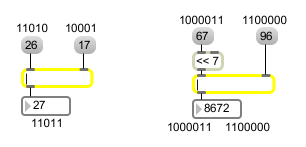# |

Bitwise union of two numbers

## Description

The | object performs a bit-by-bit "or" of two numbers (expressed in binary for the task). It is similar to the operator found in the C programming language.

## Arguments

Name Type Opt Description
initial-value int opt Sets an initial value to be or-ed with a number received in the left inlet.

## Messages

 bang In left inlet: Performs the calculation with the numbers currently stored. If there is no argument, | initially holds 0. int input [int] In left inlet: Outputs a number composed of all those bits which are 1 in either of the two numbers. (inlet1) comparison-number [int] In right inlet: The number is stored for combination with a number received in the left inlet. float input [float] Converted to int. set set-input [int] In left inlet: The word set followed by a number will set the input to the bitwise-or operation without causing output (a successive bang will output the result). list input [list] In left inlet: Combines the first and second numbers bit-by-bit, and outputs a number composed of all those bits which are 1 in either of the two numbers.

## Output

int: All the non-zero bits of the two numbers received in the inlets are combined. If a bit is 1 in either one of the numbers, it will be 1 in the output number, otherwise it will be 0 in the output number.

## ExamplesAll non-zero bits are combined......... Can be used to pack two numbers into one int